Hello, Guest!
 TITLE : Unit 09: Geometry – Points, Lines, and Angles SUGGESTED DURATION : 11 days

#### Unit Overview

Introduction
This unit bundles student expectations that address identifying basic elements of geometry, lines of symmetry, and the classification of two-dimensional figures based on properties and attributes. According to the Texas Education Agency, mathematical process standards including application, tools, communication, representations, relationships, and justifications should be integrated (when applicable) with content knowledge and skills so that students are prepared to use mathematics in everyday life, society, and the workplace.

Prior to this Unit
In Grade 3, students used attributes to recognize rhombuses, parallelograms, trapezoids, rectangles, and squares as examples of quadrilaterals and drew examples of quadrilaterals that do not belong to any of these subcategories. Students also classified and sorted two- and three-dimensional figures, including cones, cylinders, spheres, triangular and rectangular prisms, and cubes, based on attributes using formal geometric language. In Grade 4 Unit 08, students identified parallel and perpendicular lines and used these attributes to develop the formulas for perimeter and area of rectangles and squares.

During this Unit
Students examine the foundations of geometry by identifying points, lines, line segments, rays, angles, and perpendicular and parallel lines. These concepts are essential for the ability to classify two-dimensional figures based on the presence or absence of parallel or perpendicular lines or the presence or absence of angles of a specified size. Although students have recognized examples of quadrilaterals in previous grade levels, students are expected to use formal geometric language such as parallel, perpendicular, acute, obtuse, and right angle to classify two-dimensional figures. Additionally, students apply knowledge of right angles to identify acute, right, and obtuse triangles. Symmetry is explored within two-dimensional figures as students identify and draw one or more lines of symmetry, if they exist, for two-dimensional figures.

Other considerations: Reference the Mathematics COVID-19 Implementation Tool Grade 4

After this Unit
In Unit 10, students will closely examine angles as they measure angles in degrees using a protractor and draw angles of a given measure. They will also determine the measure of an unknown angle formed by two non-overlapping adjacent angles given one or both angle measures. In Grade 5, students will classify two-dimensional figures based on their attributes and properties in a hierarchy of sets and subsets using graphic organizers.

In Grade 4, identifying  points, lines, line segments, rays, angles, and perpendicular and parallel lines as well as identifying and drawing one or more lines of symmetry, if they exist, for a two-dimensional figure are STAAR Supporting Standards 4.6A and 4.6B. These standards are part of the Grade 4 Texas Response to Curriculum Focal Points (TxRCFP): Grade Level Connections. Applying  knowledge of right angles to identify acute, right, and obtuse triangles is STAAR Supporting Standard 4.6C, while classifying two-dimensional figures based on the presence or absence of parallel or perpendicular lines or the presence or absence of angles of a specified size is STAAR Readiness Standard 4.6D. Both of these standards, along with STAAR Supporting Standard 4.6A are part of the Grade 4 Focal Point: Measuring Angles (TxRCFP). All of the standards in this unit are included in Grade 4 STAAR Reporting Category 3: Geometry and Measurement. This unit is supporting the development of the Texas College and Career Readiness Standards (TxCCRS): I. Numeric Reasoning B1; II. Algebraic Reasoning D1, D2; III. Geometric and Spatial Reasoning A1, B1; V. Statistical Reasoning A1, C2; VII. Problem Solving and Reasoning A1, A2, A3, A4, A5, B1, C1, D1, D2; VIII. Communication and Representation A1, A2, A3, B1, B2, C1, C2, C3; IX. Connections A1, A2, B1, B2, B3.

Research
According to Van de Walle and Lovin (2006), “Children need experiences with a rich variety of both two- and three-dimensional shapes. It is useful for students to be able to identify common shapes, notice likenesses and differences among shapes, become aware of the properties that different shapes have, and eventually use these properties to further define and understand their geometric world. As students find out more about shapes over time, they can begin to appreciate how definitions of special shapes come to be” (p. 212). The National Council of Teachers of Mathematics (2000) concludes that the development of geometric concepts should take “a broader view of the power of geometry by calling on students to analyze characteristics of geometric shapes and make mathematical arguments about the geometric relationship, as well as to use visualization, spatial reasoning, and geometric modeling to solve problems. Geometry is a natural area of mathematics for the development of students’ reasoning and justification skills” (p. 3).

National Council of Teachers of Mathematics. (2000). Executive summary: Principles and standards for school mathematics. Reston, VA: National Council of Teachers of Mathematics, Inc.
Texas Education Agency & Texas Higher Education Coordinating Board. (2009). Texas college and career readiness standards. Retrieved from http://www.thecb.state.tx.us/index.cfm?objectid=E21AB9B0-2633-11E8-BC500050560100A9
Texas Education Agency. (2013). Texas response to curriculum focal points for kindergarten through grade 8 mathematics. Retrieved from https://www.texasgateway.org/resource/txrcfp-texas-response-curriculum-focal-points-k-8-mathematics-revised-2013
Van de Walle, J., & Lovin, L. (2006). Teaching student-centered mathematics grades 3 – 5. Boston, MA: Pearson Education, Inc.

 Geometric, spatial, and measurement reasoning are foundational to visualizing, analyzing, and applying relationships within and between scale, shapes, quantities, and spatial relations in everyday life. Why is developing geometric, spatial, and measurement reasoning essential? How does geometric, spatial, and measurement reasoning affect how one sees and works in the world?
Unit Understandings
and Questions
Overarching Concepts
and Unit Concepts
Performance Assessment(s)
• Illustrating and analyzing geometric relationships in models and diagrams aid in representing the attributes of geometric figures with quantifiable measures in order to generalize geometric relationships and solve problem situations.
• What attributes and properties exist in two-dimensional figures?
• How can illustrations and/or symbols aid in identifying …
• points?
• lines?
• line segments?
• rays?
• angles?
• parallel lines?
• perpendicular lines?
• How are angles classified?
• What relationships …
• exist
• do not exist
… within and between points, lines, line segments, rays, angles, parallel lines, and perpendicular lines?
• What strategies can be used to …
• identify
• draw
… one or more lines of symmetry for a two-dimensional figure?
• What common characteristics exist in two-dimensional figures that have …
• one
• more than one
• no
… line(s) of symmetry?
• Where are lines of symmetry found in the real-world?
• What relationships exist between right angles and …
• right triangles?
• acute triangles?
• obtuse triangles?
• How is the presence or absence of …
• parallel lines
• perpendicular lines
• angles of a specified size
… used to classify two-dimensional figures?
• How can a two-dimensional figure be classified in more than one way?
• Geometry
• Geometric Attributes and Properties
• Classification
• Geometric Representations
• One-dimensional representations
• Two-dimensional figures
• Symmetry
• Measurement
• Geometric Relationships
• Angles
• Associated Mathematical Processes
• Application
• Problem Solving Model
• Tools and Techniques
• Communication
• Representations
• Relationships
• Justification
 Assessment information provided within the TEKS Resource System are examples that may, or may not, be used by your child’s teacher. In accordance with section 26.006 (2) of the Texas Education Code, "A parent is entitled to review each test administered to the parent’s child after the test is administered." For more information regarding assessments administered to your child, please visit with your child’s teacher.

#### MISCONCEPTIONS / UNDERDEVELOPED CONCEPTS

Misconceptions:

• Students may confuse the notation for lines, line segments, and rays.
• Students might use the terms parallel and perpendicular interchangeably rather than making a connection between the terms and the attributes they represent.
• Students may misunderstand that notations for points, lines, and rays are only representations of abstract ideas and are not actual objects.
• Students may refer to a square or rhombus as a diamond.
• Some students may not associate the line of symmetry as the line of reflection.

Underdeveloped Concepts:

• Students may understand that many shapes fall under the classification of quadrilaterals, but may not know how the name of each quadrilateral depends on its attributes.
• Some students may think that two-dimensional figures are only polygons.

#### Unit Vocabulary

• Acute angle – an angle that measures less than 90°
• Acute triangle – a triangle in which each of the three angles is acute (less than 90°)
• Adjacent angles – two non-overlapping angles that share a common vertex and exactly one ray
• Angle – two rays with a common endpoint (the vertex)
• Angle congruency marks – angle marks indicating angles of the same measure
• Classify – applying an attribute to categorize a sorted group
• Congruent – of equal measure, having exactly the same size and same shape
• Degree – the measure of an angle where each degree representsof a circle
• Equiangular – all angles in a polygon are congruent in measure
• Equilateral – all side lengths of a polygon are congruent in measure
• Intersecting lines – lines that meet or cross at a point
• Irregular figure – a polygon with sides and/or angles that are not all congruent
• Line – a set of points that form a straight path that goes in opposite directions without ending
• Line of symmetry – line dividing an image into two congruent parts that are mirror images of each other
• Line segment – part of a line between two points on the line, called endpoints of the segment
• Obtuse angle – an angle that measures greater than 90° but less than 180°
• Obtuse triangle – a triangle that has one obtuse angle (greater than 90°) and two acute angles
• Parallel lines – lines that lie in the same plane, never intersect, and are always the same distance apart
• Perpendicular lines – lines that intersect at right angles to each other to form square corners
• Point – a specific location in space
• Polygon – a closed figure with at least 3 sides, where all sides are straight (no curves)
• Ray – part of a line that begins at one endpoint and continues without end in one direction
• Regular figure – a polygon with all sides and angles congruent
• Right angle – an angle (formed by perpendicular lines) that measures exactly 90°
• Right triangle – a triangle with one right angle (exactly 90°) and two acute angles
• Side congruency marks – side marks indicating side lengths of the same measure
• Straight angle – an angle that measures 180° (a straight line)
• Triangle – a polygon with three sides and three vertices
• Two-dimensional figure – a figure with two basic units of measure, usually length and width

Related Vocabulary:

 7-gon (heptagon) 9-gon (nonagon) 11-gon (hendecagon) 12-gon (dodecagon) Circle Decagon Diagonal Equilateral triangle Hexagon Horizontal Isosceles trapezoid Isosceles triangle Label Line of reflection Octagon Parallelogram Pentagon Quadrilateral Rectangle Rhombus Right trapezoid Scalene triangle Side Square Trapezoid Unit Vertex Vertical
Unit Assessment Items System Resources Other Resources

Show this message:

Unit Assessment Items that have been published by your district may be accessed through Search All Components in the District Resources tab. Assessment items may also be found using the Assessment Center if your district has granted access to that tool.

System Resources may be accessed through Search All Components in the District Resources Tab.

Texas Higher Education Coordinating Board – Texas College and Career Readiness Standards

Texas Education Agency – Texas Response to Curriculum Focal Points for K-8 Mathematics Revised 2013

Texas Education Agency – Mathematics Curriculum

Texas Education Agency – STAAR Mathematics Resources

Texas Education Agency Texas Gateway – Revised Mathematics TEKS: Vertical Alignment Charts

Texas Education Agency Texas Gateway – Mathematics TEKS: Supporting Information

Texas Education Agency Texas Gateway – Interactive Mathematics Glossary

Texas Education Agency Texas Gateway – Resources Aligned to Grade 4 Mathematics TEKS

TAUGHT DIRECTLY TEKS

TEKS intended to be explicitly taught in this unit.

TEKS/SE Legend:

• Knowledge and Skills Statements (TEKS) identified by TEA are in italicized, bolded, black text.
• Student Expectations (TEKS) identified by TEA are in bolded, black text.
• Student Expectations (TEKS) are labeled Readiness as identified by TEA of the assessed curriculum.
• Student Expectations (TEKS) are labeled Supporting as identified by TEA of the assessed curriculum.
• Student Expectations (TEKS) are labeled Process standards as identified by TEA of the assessed curriculum.
• Portions of the Student Expectations (TEKS) that are not included in this unit but are taught in previous or future units are indicated by a strike-through.

Specificity Legend:

• Supporting information / clarifications (specificity) written by TEKS Resource System are in blue text.
• Unit-specific clarifications are in italicized, blue text.
• Information from Texas Education Agency (TEA), Texas College and Career Readiness Standards (TxCCRS), Texas Response to Curriculum Focal Points (TxRCFP) is labeled.
• A Partial Specificity label indicates that a portion of the specificity not aligned to this unit has been removed.
TEKS# SE# TEKS SPECIFICITY
4.1 Mathematical process standards. The student uses mathematical processes to acquire and demonstrate mathematical understanding. The student is expected to:
4.1A Apply mathematics to problems arising in everyday life, society, and the workplace.
Process Standard

Apply

MATHEMATICS TO PROBLEMS ARISING IN EVERYDAY LIFE, SOCIETY, AND THE WORKPLACE

Including, but not limited to:

• Mathematical problem situations within and between disciplines
• Everyday life
• Society
• Workplace

Note(s):

• The mathematical process standards may be applied to all content standards as appropriate.
• TxRCFP:
• Developing fluency with efficient use of the four arithmetic operations on whole numbers and using this knowledge to solve problems
• Measuring angles
• Understanding decimals and addition and subtraction of decimals
• Building foundations for addition and subtraction of fractions
• TxCCRS:
• VII.D. Problem Solving and Reasoning – Real-world problem solving
• VII.D.1. Interpret results of the mathematical problem in terms of the original real-world situation.
• IX.A. Connections – Connections among the strands of mathematics
• IX.A.1. Connect and use multiple key concepts of mathematics in situations and problems.
• IX.A.2. Connect mathematics to the study of other disciplines.
• IX.B. Connections – Connections of mathematics to nature, real-world situations, and everyday life
• IX.B.1. Use multiple representations to demonstrate links between mathematical and real-world situations.
• IX.B.2. Understand and use appropriate mathematical models in the natural, physical, and social sciences.
• IX.B.3. Know and understand the use of mathematics in a variety of careers and professions.
4.1B Use a problem-solving model that incorporates analyzing given information, formulating a plan or strategy, determining a solution, justifying the solution, and evaluating the problem-solving process and the reasonableness of the solution.
Process Standard

Use

A PROBLEM-SOLVING MODEL THAT INCORPORATES ANALYZING GIVEN INFORMATION, FORMULATING A PLAN OR STRATEGY, DETERMINING A SOLUTION, JUSTIFYING THE SOLUTION, AND EVALUATING THE PROBLEM-SOLVING PROCESS AND THE REASONABLENESS OF THE SOLUTION

Including, but not limited to:

• Problem-solving model
• Analyze given information
• Formulate a plan or strategy
• Determine a solution
• Justify the solution
• Evaluate the problem-solving process and the reasonableness of the solution

Note(s):

• The mathematical process standards may be applied to all content standards as appropriate.
• TxRCFP:
• Developing fluency with efficient use of the four arithmetic operations on whole numbers and using this knowledge to solve problems
• Measuring angles
• Understanding decimals and addition and subtraction of decimals
• Building foundations for addition and subtraction of fractions
• TxCCRS:
• I.B. Numeric Reasoning – Number sense and number concepts
• I.B.1. Use estimation to check for errors and reasonableness of solutions.
• V.A. Statistical Reasoning – Design a study
• V.A.1. Formulate a statistical question, plan an investigation, and collect data.
• VII.A. Problem Solving and Reasoning – Mathematical problem solving
• VII.A.1. Analyze given information.
• VII.A.2. Formulate a plan or strategy.
• VII.A.3. Determine a solution.
• VII.A.4. Justify the solution.
• VII.A.5. Evaluate the problem-solving process.
• VII.D. Problem Solving and Reasoning – Real-world problem solving
• VII.D.2. Evaluate the problem-solving process.
4.1C

Select tools, including real objects, manipulatives, paper and pencil, and technology as appropriate, and techniques, including mental math, estimation, and number sense as appropriate, to solve problems.

Process Standard

Select

TOOLS, INCLUDING REAL OBJECTS, MANIPULATIVES, PAPER AND PENCIL, AND TECHNOLOGY AS APPROPRIATE TO SOLVE PROBLEMS

Including, but not limited to:

• Appropriate selection of tool(s) to apply in order to solve problems
• Tools
• Real objects
• Manipulatives
• Paper and pencil
• Technology

Note(s):

• The mathematical process standards may be applied to all content standards as appropriate.
• TxRCFP:
• Developing fluency with efficient use of the four arithmetic operations on whole numbers and using this knowledge to solve problems
• Measuring angles
• Understanding decimals and addition and subtraction of decimals
• Building foundations for addition and subtraction of fractions
• TxCCRS:
• I.B. Numeric Reasoning – Number sense and number concepts
• I.B.1. Use estimation to check for errors and reasonableness of solutions.
• V.C. Statistical Reasoning – Analyze, interpret, and draw conclusions from data
• V.C.2. Analyze relationships between paired data using spreadsheets, graphing calculators, or statistical software.
4.1D

Communicate mathematical ideas, reasoning, and their implications using multiple representations, including symbols, diagrams, graphs, and language as appropriate.

Process Standard

Communicate

MATHEMATICAL IDEAS, REASONING, AND THEIR IMPLICATIONS USING MULTIPLE REPRESENTATIONS, INCLUDING SYMBOLS, DIAGRAMS, AND LANGUAGE AS APPROPRIATE

Including, but not limited to:

• Mathematical ideas, reasoning, and their implications
• Multiple representations, as appropriate
• Symbols
• Diagrams
• Language

Note(s):

• The mathematical process standards may be applied to all content standards as appropriate.
• TxRCFP:
• Developing fluency with efficient use of the four arithmetic operations on whole numbers and using this knowledge to solve problems
• Measuring angles
• Understanding decimals and addition and subtraction of decimals
• Building foundations for addition and subtraction of fractions
• TxCCRS:
• II.D. Algebraic Reasoning – Representing relationships
• II.D.1. Interpret multiple representations of equations, inequalities, and relationships.
• II.D.2. Convert among multiple representations of equations, inequalities, and relationships.
• VIII.A. Communication and Representation – Language, terms, and symbols of mathematics
• VIII.A.1. Use mathematical symbols, terminology, and notation to represent given and unknown information in a problem.
• VIII.A.2. Use mathematical language to represent and communicate the mathematical concepts in a problem.
• VIII.A.3. Use mathematical language for reasoning, problem solving, making connections, and generalizing.
• VIII.B. Communication and Representation – Interpretation of mathematical work
• VIII.B.1. Model and interpret mathematical ideas and concepts using multiple representations.
• VIII.B.2. Summarize and interpret mathematical information provided orally, visually, or in written form within the given context.
• VIII.C. Communication and Representation – Presentation and representation of mathematical work
• VIII.C.1. Communicate mathematical ideas, reasoning, and their implications using symbols, diagrams, models, graphs, and words.
• VIII.C.2. Create and use representations to organize, record, and communicate mathematical ideas.
• VIII.C.3. Explain, display, or justify mathematical ideas and arguments using precise mathematical language in written or oral communications.
• IX.B. Connections – Connections of mathematics to nature, real-world situations, and everyday life
• IX.B.1. Use multiple representations to demonstrate links between mathematical and real-world situations.
4.1E Create and use representations to organize, record, and communicate mathematical ideas.
Process Standard

Create, Use

REPRESENTATIONS TO ORGANIZE, RECORD, AND COMMUNICATE MATHEMATICAL IDEAS

Including, but not limited to:

• Representations of mathematical ideas
• Organize
• Record
• Communicate
• Evaluation of the effectiveness of representations to ensure clarity of mathematical ideas being communicated
• Appropriate mathematical vocabulary and phrasing when communicating mathematical ideas

Note(s):

• The mathematical process standards may be applied to all content standards as appropriate.
• TxRCFP:
• Developing fluency with efficient use of the four arithmetic operations on whole numbers and using this knowledge to solve problems
• Measuring angles
• Understanding decimals and addition and subtraction of decimals
• Building foundations for addition and subtraction of fractions
• TxCCRS:
• VIII.B. Communication and Representation – Interpretation of mathematical work
• VIII.B.1. Model and interpret mathematical ideas and concepts using multiple representations.
• VIII.B.2. Summarize and interpret mathematical information provided orally, visually, or in written form within the given context.
• VIII.C. Communication and Representation – Presentation and representation of mathematical work
• VIII.C.1. Communicate mathematical ideas, reasoning, and their implications using symbols, diagrams, models, graphs, and words.
• VIII.C.2. Create and use representations to organize, record, and communicate mathematical ideas.
4.1F Analyze mathematical relationships to connect and communicate mathematical ideas.
Process Standard

Analyze

MATHEMATICAL RELATIONSHIPS TO CONNECT AND COMMUNICATE MATHEMATICAL IDEAS

Including, but not limited to:

• Mathematical relationships
• Connect and communicate mathematical ideas
• Conjectures and generalizations from sets of examples and non-examples, patterns, etc.
• Current knowledge to new learning

Note(s):

• The mathematical process standards may be applied to all content standards as appropriate.
• TxRCFP:
• Developing fluency with efficient use of the four arithmetic operations on whole numbers and using this knowledge to solve problems
• Measuring angles
• Understanding decimals and addition and subtraction of decimals
• Building foundations for addition and subtraction of fractions
• TxCCRS:
• VII.A. Problem Solving and Reasoning – Mathematical problem solving
• VII.A.1. Analyze given information.
• VIII.A. Communication and Representation – Language, terms, and symbols of mathematics
• VIII.A.1. Use mathematical symbols, terminology, and notation to represent given and unknown information in a problem.
• VIII.A.2. Use mathematical language to represent and communicate the mathematical concepts in a problem.
• VIII.A.3. Use mathematical language for reasoning, problem solving, making connections, and generalizing.
• VIII.B. Communication and Representation – Interpretation of mathematical work
• VIII.B.1. Model and interpret mathematical ideas and concepts using multiple representations.
• VIII.C. Communication and Representation – Presentation and representation of mathematical work
• VIII.C.1. Communicate mathematical ideas, reasoning, and their implications using symbols, diagrams, models, graphs, and words.
• VIII.C.2. Create and use representations to organize, record, and communicate mathematical ideas.
• VIII.C.3. Explain, display, or justify mathematical ideas and arguments using precise mathematical language in written or oral communications.
• IX.A. Connections – Connections among the strands of mathematics
• IX.A.1. Connect and use multiple key concepts of mathematics in situations and problems.
• IX.A.2. Connect mathematics to the study of other disciplines.
4.1G Display, explain, and justify mathematical ideas and arguments using precise mathematical language in written or oral communication.
Process Standard

Display, Explain, Justify

MATHEMATICAL IDEAS AND ARGUMENTS USING PRECISE MATHEMATICAL LANGUAGE IN WRITTEN OR ORAL COMMUNICATION

Including, but not limited to:

• Mathematical ideas and arguments
• Validation of conclusions
• Displays to make work visible to others
• Diagrams, visual aids, written work, etc.
• Explanations and justifications
• Precise mathematical language in written or oral communication

Note(s):

• The mathematical process standards may be applied to all content standards as appropriate.
• TxRCFP:
• Developing fluency with efficient use of the four arithmetic operations on whole numbers and using this knowledge to solve problems
• Measuring angles
• Understanding decimals and addition and subtraction of decimals
• Building foundations for addition and subtraction of fractions
• TxCCRS:
• VII.A. Problem Solving and Reasoning – Mathematical problem solving
• VII.A.4. Justify the solution.
• VII.B. Problem Solving and Reasoning – Proportional reasoning
• VII.B.1. Use proportional reasoning to solve problems that require fractions, ratios, percentages, decimals, and proportions in a variety of contexts using multiple representations.
• VII.C. Problem Solving and Reasoning – Logical reasoning
• VII.C.1. Develop and evaluate convincing arguments.
• VIII.A. Communication and Representation – Language, terms, and symbols of mathematics
• VIII.A.3. Use mathematical language for reasoning, problem solving, making connections, and generalizing.
• VIII.B. Communication and Representation – Interpretation of mathematical work
• VIII.B.1. Model and interpret mathematical ideas and concepts using multiple representations.
• VIII.B.2. Summarize and interpret mathematical information provided orally, visually, or in written form within the given context.
• VIII.C. Communication and Representation – Presentation and representation of mathematical work
• VIII.C.3. Explain, display, or justify mathematical ideas and arguments using precise mathematical language in written or oral communications.
4.6 Geometry and measurement. The student applies mathematical process standards to analyze geometric attributes in order to develop generalizations about their properties. The student is expected to:
4.6A Identify points, lines, line segments, rays, angles, and perpendicular and parallel lines.
Supporting Standard

Identify

POINTS, LINES, LINE SEGMENTS, RAYS, ANGLES, AND PERPENDICULAR AND PARALLEL LINES

Including, but not limited to:

• Point – a specific location in space
• Has no dimension and is usually represented by a small dot
• Line – a set of points that form a straight path that goes in opposite directions without ending
• Line labels
• Lines named according to two points on a line
• Lines named by one lower case cursive letter
• Parallel lines – lines that lie in the same plane, never intersect, and are always the same distance apart
• Various orientations including vertical, horizontal, diagonal, and parallel lines of even, uneven, or off-set lengths
• Notation may be given using chevrons or arrows to represent parallel lines.
• If more than one set of parallel lines are present, the number of chevrons or arrows distinguishes the sets of parallel lines.
• Intersecting lines – lines that meet or cross at a point
• Various orientations including vertical, horizontal, diagonal, and intersecting lines of even, uneven, or off-set lengths
• Perpendicular lines – lines that intersect at right angles to each other to form square corners
• Various orientations including vertical, horizontal, diagonal, and perpendicular lines of even, uneven, or off-set lengths
• Notation is given as a box in the angle corner to represent a 90° angle.
• Lines in pictorial models and polygons
• Extending lines beyond pictorial models
• Line segment – part of a line between two points on the line, called endpoints of the segment
• Ray – part of a line that begins at one endpoint and continues without end in one direction
• Relationships between line segments, rays, and lines
• A line segment is part of a ray and part of a line
• A ray is part of a line
• Degree – the measure of an angle where each degree representsof a circle
• Unit measure labels as “degrees” or with symbol for degrees (°)
• Angle – two rays with a common endpoint (the vertex)
• Angle labels for a single angle
• Angle with one letter, the letter of the vertex
• Angle label with three letters, where the middle letter is the vertex of the angle
• Angle label with a number or letter designated within the angle
• Angle symbol with one letter, the letter of the vertex
• Angle symbol with three letters, where the middle letter is the vertex of the angle
• Angle symbol with a number or letter designated within the angle
• Angle labels for adjacent angles
• Adjacent angles – two non-overlapping angles that share a common vertex and exactly one ray
• Various angle types/names
• Right angle, 90°, used as a benchmark to identify and name angles
• Acute angle – an angle that measures less than 90°
• Right angle – an angle (formed by perpendicular lines) that measures exactly 90°
• Notation is given as a box in the angle corner to represent a 90° angle.
• Obtuse angle – an angle that measures greater than 90° but less than 180°
• Straight angle – an angle that measures 180° (a straight line)
• Angles in pictorial models and polygons

Note(s):

• Grade 3 used attributes to recognize rhombuses, parallelograms, trapezoids, rectangles, and squares as examples of quadrilaterals and drew examples of quadrilaterals that do not belong to any of these subcategories.
• Various mathematical process standards will be applied to this student expectation as appropriate.
• TxRCFP:
• Measuring angles
• Grade Level Connections (reinforces previous learning and/or provides development for future learning)
• TxCCRS:
• III.A. Geometric and Spatial Reasoning – Figures and their properties
• III.A.1. Recognize characteristics and dimensional changes of two- and three-dimensional figures.
4.6B Identify and draw one or more lines of symmetry, if they exist, for a two-dimensional figure.
Supporting Standard

Identify, Draw

ONE OR MORE LINES OF SYMMETRY, IF THEY EXIST, FOR A TWO-DIMENSIONAL FIGURE

Including, but not limited to:

• Line of symmetry – line dividing an image into two congruent parts that are mirror images of each other
• Two-dimensional figure – a figure with two basic units of measure, usually length and width
• Two-dimensional figures and real-world figures
• Shapes with more than one line of symmetry
• Shapes with no lines of symmetry
• Shapes on which lines of symmetry have not been drawn
• Across a vertical line, across a horizontal line, or across a diagonal line of symmetry
• A line of reflection exists for a figure if for every point on one side of the line of reflection, there is a corresponding point the same distance from the line.

Note(s):

• Grade 3 used attributes to recognize rhombuses, parallelograms, trapezoids, rectangles, and squares as examples of quadrilaterals and drew examples of quadrilaterals that do not belong to any of these subcategories.
• Various mathematical process standards will be applied to this student expectation as appropriate.
• TxRCFP:
• Grade Level Connections (reinforces previous learning and/or provides development for future learning)
• TxCCRS:
• III.A. Geometric and Spatial Reasoning – Figures and their properties
• III.A.1. Recognize characteristics and dimensional changes of two- and three-dimensional figures.
• III.B. Geometric and Spatial Reasoning – Transformations and symmetry
• III.B.1. Identify transformations and symmetries of figures.
4.6C Apply knowledge of right angles to identify acute, right, and obtuse triangles.
Supporting Standard

Apply

KNOWLEDGE OF RIGHT ANGLES TO IDENTIFY ACUTE, RIGHT, AND OBTUSE TRIANGLES

Including, but not limited to:

• Angle – two rays with a common endpoint (the vertex)
• Various angle types/names
• Right angle, 90°, used as a benchmark to identify and name angles
• Acute angle – an angle that measures less than 90°
• Right angle – an angle (formed by perpendicular lines) that measures exactly 90°
• Notation is given as a box in the angle corner to represent a 90° angle.
• Obtuse angle – an angle that measures greater than 90° but less than 180°
• Triangle – a polygon with three sides and three vertices
• Triangles are named based on their largest angle.
• Acute triangle – a triangle in which each of the three angles is acute (less than 90°)
• Right triangle – a triangle with one right angle (exactly 90°) and two acute angles
• Obtuse triangle – a triangle that has one obtuse angle (greater than 90°) and two acute angles

Note(s):

• Grade 3 used attributes to recognize rhombuses, parallelograms, trapezoids, rectangles, and squares as examples of quadrilaterals and drew examples of quadrilaterals that do not belong to any of these subcategories.
• Grade 4 introduces formal and symbolic geometric language for lines, line segments, rays, and angles.
• Grade 5 will classify two-dimensional figures in a hierarchy of sets and subsets using graphic organizers based on their attributes and properties.
• Various mathematical process standards will be applied to this student expectation as appropriate.
• TxRCFP:
• Measuring angles
• TxCCRS:
• III.A. Geometric and Spatial Reasoning – Figures and their properties
• III.A.1. Recognize characteristics and dimensional changes of two- and three-dimensional figures.
4.6D Classify two-dimensional figures based on the presence or absence of parallel or perpendicular lines or the presence or absence of angles of a specified size.

Classify

TWO-DIMENSIONAL FIGURES BASED ON THE PRESENCE OR ABSENCE OF PARALLEL OR PERPENDICULAR LINES OR THE PRESENCE OR ABSENCE OF ANGLES OF A SPECIFIED SIZE

Including, but not limited to:

• Two-dimensional figure – a figure with two basic units of measure, usually length and width
• Regular figure – a polygon with all sides and angles congruent
• Irregular figure – a polygon with sides and/or angles that are not all congruent
• Classify – applying an attribute to categorize a sorted group
• Angle – two rays with a common endpoint (the vertex)
• Various angle types/names
• Right angle, 90°, used as a benchmark to identify and name angles
• Acute angle – an angle that measures less than 90°
• Right angle – an angle (formed by perpendicular lines) that measures exactly 90°
• Notation is given as a box in the angle corner to represent a 90° angle.
• Obtuse angle – an angle that measures greater than 90° but less than 180°
• Line – a set of points that form a straight path that goes in opposite directions without ending
• Parallel lines – lines that lie in the same plane, never intersect, and are always the same distance apart
•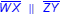means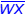is parallel to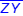.
• Notation may be given using chevrons or arrows to represent parallel lines.
• If more than one set of parallel lines are present, the number of chevrons or arrows distinguishes the sets of parallel lines.
• Perpendicular lines – lines that intersect at right angles to each other to form square corners
•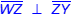means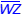is perpendicular to.
• Notation is given as a box in the angle corner to represent a 90° angle.
• Sides of two-dimensional figures are composed of line segments, the part of a line between two points on the line
• Congruent – of equal measure, having exactly the same size and same shape
• Angle congruency marks – angle marks indicating angles of the same measure
• mAmC means ∠A is congruent to ∠C.
• Side congruency marks – side marks indicating side lengths of the same measure
•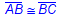means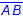is congruent to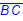.
• Equilateral – all side lengths of a polygon are congruent in measure
• Equiangular – all angles in a polygon are congruent in measure
• Types of two-dimensional figures
• Circle
• A figure formed by a closed curve with all points equal distance from the center
• No straight sides
• No vertices
• No parallel or perpendicular sides
• Polygon – a closed figure with at least 3 sides, where all sides are straight (no curves)
• Types of polygons
• Triangle
• 3 sides
• 3 vertices
• No parallel sides
• Types of triangles
• Triangles are named based on their largest angle.
• Scalene triangle
• 3 sides
• 3 vertices
• No congruent sides
• No parallel sides
• Up to one possible pair of perpendicular sides
• Right triangle with two sides that are perpendicular to form a right angle and three different side lengths
• No congruent angles
• Right triangle with one 90° angle and two other angles each of different measures
• Obtuse triangle with one angle greater than 90° and two other angles each of different measures
• Acute triangle with all angles less than 90° and all angles of different measures
• Isosceles triangle
• 3 sides
• 3 vertices
• At least 2 congruent sides
• No parallel sides
• Up to one possible pair of perpendicular sides
• Right triangle with two sides that are perpendicular to form a right angle and are each of the same length
• At least 2 congruent angles
• Right triangle with one 90° angle and two other angles each of the same measure
• Obtuse triangle with two angles of the same measure and one angle greater than 90°
• Acute triangle with all angles measuring less than 90° and at least two of the angles of the same measure
• Equilateral triangle/Equiangular triangle (a special type of isosceles triangle)
• 3 sides
• 3 vertices
• All sides congruent
• No parallel or perpendicular sides
• All angles congruent
• Acute triangle with all angles measuring 60°
• 4 sides
• 4 vertices
• Trapezoid
• 4 sides
• 4 vertices
• Exactly one pair of parallel sides
• Types of trapezoids
• Isosceles trapezoid
• 4 sides
• 4 vertices
• Exactly one pair of parallel sides
• At least 2 congruent sides, where 2 of the sides are opposite each other
• Right trapezoid
• 4 sides
• 4 vertices
• Exactly one pair of parallel sides
• 2 pairs of perpendicular sides
• 2 right angles
• Parallelogram
• 4 sides
• 4 vertices
• Opposite sides congruent
• 2 pairs of parallel sides
• Opposite angles congruent
• Types of parallelograms
• Rectangle
• 4 sides
• 4 vertices
• Opposite sides congruent
• 2 pairs of parallel sides
• 4 pairs of perpendicular sides
• 4 right angles
• Rhombus
• 4 sides
• 4 vertices
• All sides congruent
• 2 pairs of parallel sides
• Opposite angles congruent
• Square (a special type of rectangle and a special type of rhombus)
• 4 sides
• 4 vertices
• All sides congruent
• 2 pairs of parallel sides
• 4 pairs of perpendicular sides
• 4 right angles
• Pentagon
• 5 sides
• 5 vertices
• Possible parallel and/or perpendicular sides
• Possible acute, obtuse, and/or right angles
• Hexagon
• 6 sides
• 6 vertices
• Possible parallel and/or perpendicular sides
• Possible acute, obtuse, and/or right angles
• 7-gon (heptagon)
• 7 sides
• 7 vertices
• Possible parallel and/or perpendicular sides
• Possible acute, obtuse, and/or right angles
• Octagon
• 8 sides
• 8 vertices
• Possible parallel and/or perpendicular sides
• Possible acute, obtuse, and/or right angles
• 9-gon (nonagon)
• 9 sides
• 9 vertices
• Possible parallel and/or perpendicular sides
• Possible acute, obtuse, and/or right angles
• Decagon
• 10 sides
• 10 vertices
• Possible parallel and/or perpendicular sides
• Possible acute, obtuse, and/or right angles
• 11-gon (hendecagon)
• 11 sides
• 11 vertices
• Possible parallel and/or perpendicular sides
• Possible acute, obtuse, and/or right angles
• 12-gon (dodecagon)
• 12 sides
• 12 vertices
• Possible parallel and/or perpendicular sides
• Possible acute, obtuse, and/or right angles
• Classification of two-dimensional figures based on attributes of sides and angles

Note(s):